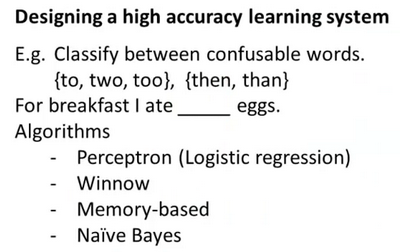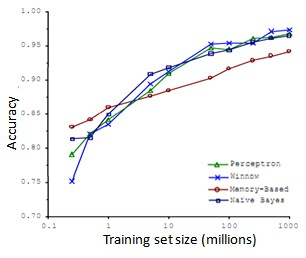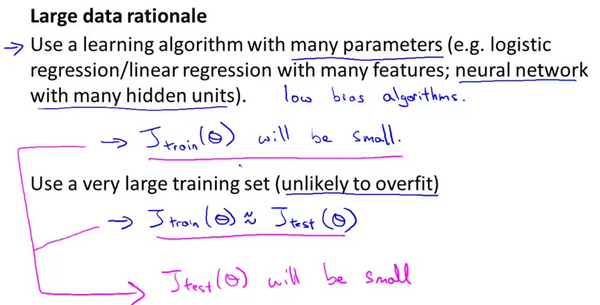# 第6周

## 十、应用机器学习的建议(Advice for Applying Machine Learning)

### 10.1 决定下一步做什么

1. 获得更多的训练样本——通常是有效的，但代价较大，下面的方法也可能有效，可考虑先采用下面的几种方法。
2. 尝试减少特征的数量
3. 尝试获得更多的特征
4. 尝试增加多项式特征
5. 尝试减少正则化程度$\lambda$
6. 尝试增加正则化程度$\lambda$

### 10.2 评估一个假设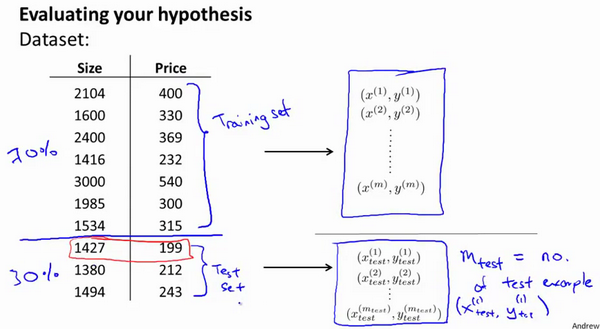1. 对于线性回归模型，我们利用测试集数据计算代价函数$J$
2. 对于逻辑回归模型，我们除了可以利用测试数据集来计算代价函数外：

$J_{test}{(\theta)} = -\frac{1}{{m}_{test}}\sum_\limits{i=1}^{m_{test}}\log{h_{\theta}(x^{(i)}_{test})}+(1-{y^{(i)}_{test}})\log{h_{\theta}(x^{(i)}_{test})}$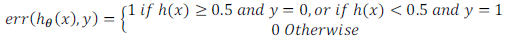### 10.3 模型选择和交叉验证集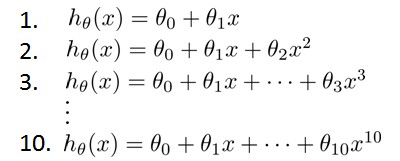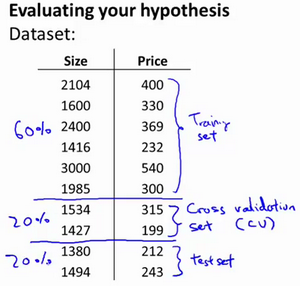1. 使用训练集训练出10个模型

2. 用10个模型分别对交叉验证集计算得出交叉验证误差（代价函数的值）

3. 选取代价函数值最小的模型

4. 用步骤3中选出的模型对测试集计算得出推广误差（代价函数的值）

Train/validation/test error

Training error:

$J_{train}(\theta) = \frac{1}{2m}\sum_\limits{i=1}^{m}(h_{\theta}(x^{(i)})-y^{(i)})^2$

Cross Validation error:

$J_{cv}(\theta) = \frac{1}{2m_{cv}}\sum_\limits{i=1}^{m}(h_{\theta}(x^{(i)}_{cv})-y^{(i)}_{cv})^2$

Test error:

$J_{test}(\theta)=\frac{1}{2m_{test}}\sum_\limits{i=1}^{m_{test}}(h_{\theta}(x^{(i)}_{cv})-y^{(i)}_{cv})^2$

### 10.4 诊断偏差和方差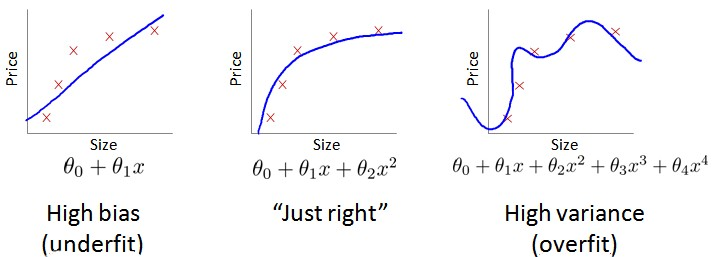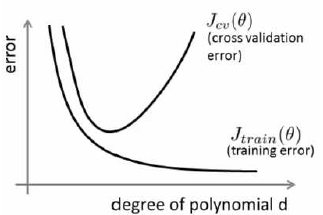Bias/variance

Training error: $J_{train}(\theta) = \frac{1}{2m}\sum_\limits{i=1}^{m}(h_{\theta}(x^{(i)})-y^{(i)})^2$

Cross Validation error: $J_{cv}(\theta) = \frac{1}{2m_{cv}}\sum_\limits{i=1}^{m}(h_{\theta}(x^{(i)}_{cv})-y^{(i)}_{cv})^2$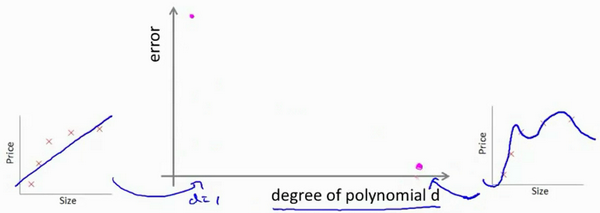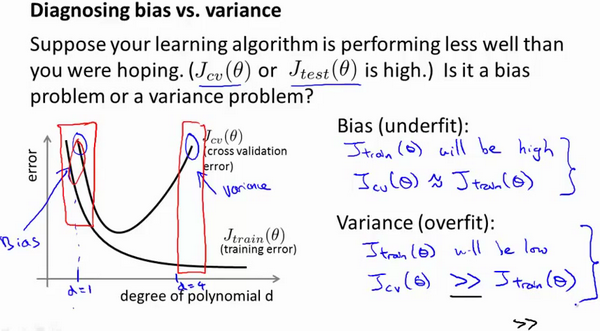### 10.5 正则化和偏差/方差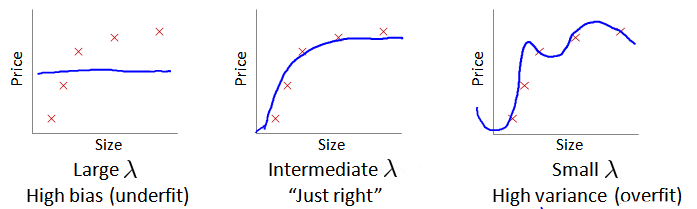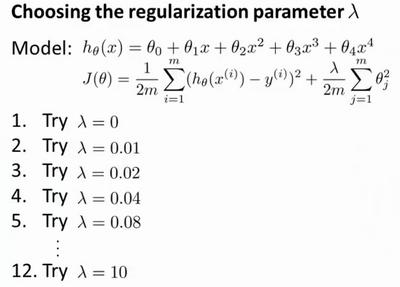1. 使用训练集训练出12个不同程度正则化的模型
2. 用12个模型分别对交叉验证集计算的出交叉验证误差
3. 选择得出交叉验证误差最小的模型
4. 运用步骤3中选出模型对测试集计算得出推广误差，我们也可以同时将训练集和交叉验证集模型的代价函数误差与λ的值绘制在一张图表上：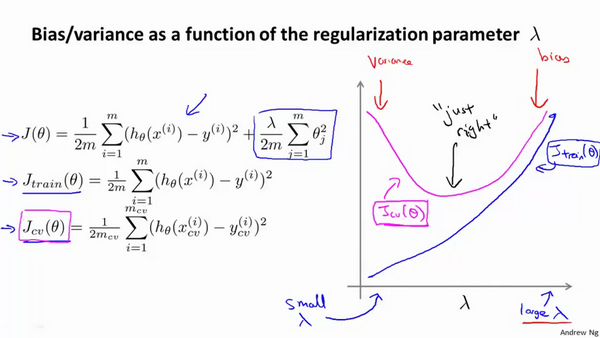• 当 $\lambda$ 较小时，训练集误差较小（过拟合）而交叉验证集误差较大 • 随着 $\lambda$ 的增加，训练集误差不断增加（欠拟合），而交叉验证集误差则是先减小后增加

### 10.6 学习曲线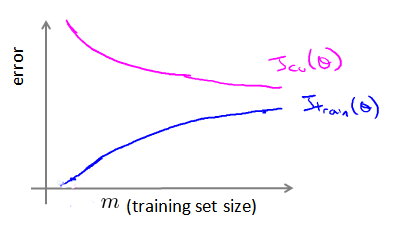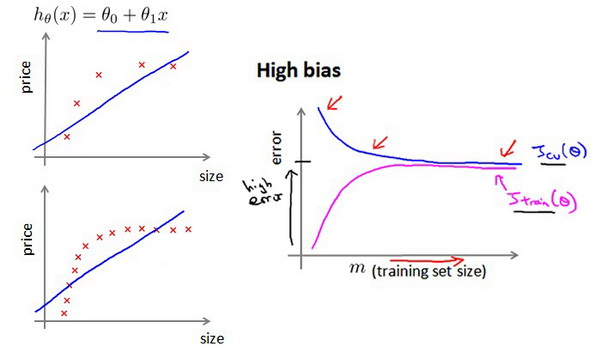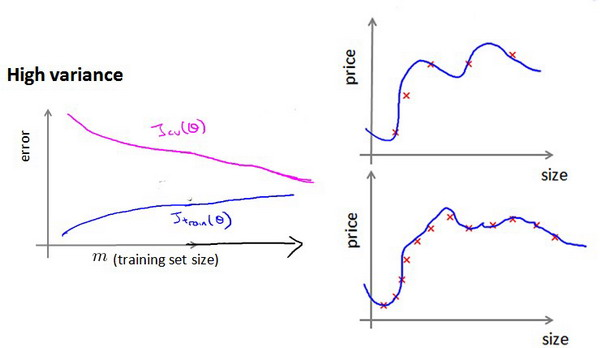### 10.7 决定下一步做什么

1. 获得更多的训练样本——解决高方差
2. 尝试减少特征的数量——解决高方差
3. 尝试获得更多的特征——解决高偏差
4. 尝试增加多项式特征——解决高偏差
5. 尝试减少正则化程度λ——解决高偏差
6. 尝试增加正则化程度λ——解决高方差

## 十一、机器学习系统的设计(Machine Learning System Design)

### 11.1 首先要做什么

1. 收集更多的数据，让我们有更多的垃圾邮件和非垃圾邮件的样本
2. 基于邮件的路由信息开发一系列复杂的特征
3. 基于邮件的正文信息开发一系列复杂的特征，包括考虑截词的处理
4. 为探测刻意的拼写错误（把watch 写成w4tch）开发复杂的算法

### 11.2 误差分析

1. 从一个简单的能快速实现的算法开始，实现该算法并用交叉验证集数据测试这个算法

2.绘制学习曲线，决定是增加更多数据，或者添加更多特征，还是其他选择 3.进行误差分析：人工检查交叉验证集中我们算法中产生预测误差的样本，看看这些样本是否有某种系统化的趋势

### 11.3 类偏斜的误差度量

1. 正确肯定True Positive,TP）：预测为真，实际为真

2.正确否定True Negative,TN）：预测为假，实际为假 3.错误肯定False Positive,FP）：预测为真，实际为假 4.错误否定False Negative,FN）：预测为假，实际为真

### 11.4 查准率和查全率之间的权衡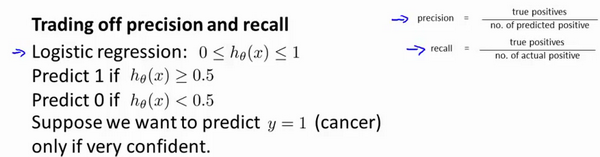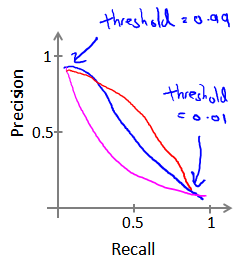${{F}_{1}}Score:2\frac{PR}{P+R}$

### 11.5 机器学习的数据